5G/NR - Frame Structure                                                          Home : www.sharetechnote.com

Frame Structure

There has been long long discussions on frame structure both in academia and in 3GPP and now we have pretty clear agreements on what a NR(5G) radio frame would look like. In this page, I will describe on NR Frame Structure that is specified in 3GPP specification (38.211). If you are intrested in those long discussions and histories about show these specification came out for your personal interests and study, refer to 5G Frame Structure Candidate page.

 Numerology - Subcarrier Spacing Numerology - Sampling Time Radio Frame Structure Slot Format Resource Grid

Numerology - Subcarrier Spacing

Comparing to LTE numberology (subcarrier spacing and symbol length), the most outstanding diffrence you can notice is thet NR support multiple different types of subcarrier spacing (in LTE there is only one type of subcarrier spacing, 15 Khz). The types NR numerology is summarized in 38.211 and I converted the table into illustration to give you intuitive understanding of these numerology.

As you see here, each numerology is labled as a parameter(u, mu in Greek). The numerology (u = 0) represents 15 kHz which is same as LTE. And as you see in the second column the subcarrier spacing of other u is derived from (u=0) by scaling up in the power of 2.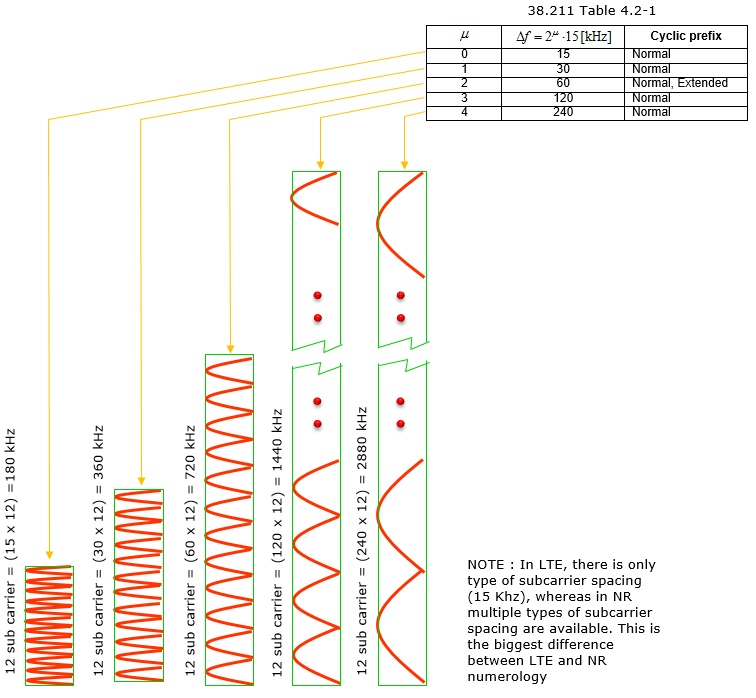Numerology and Slot Length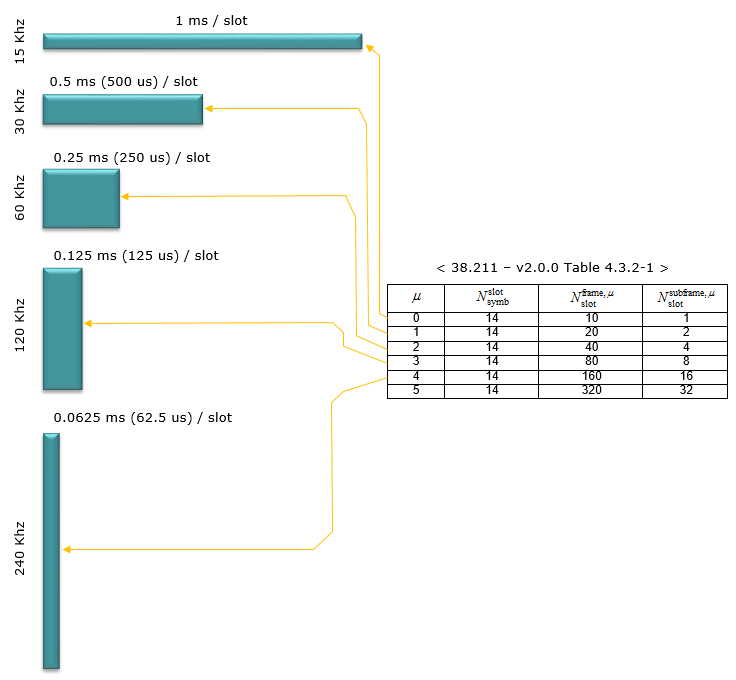Numerology - Sampling Time

Based on 38.211 - 4. Frame structure and physical resources, the sampling time (unit time) is assumed to be as follows.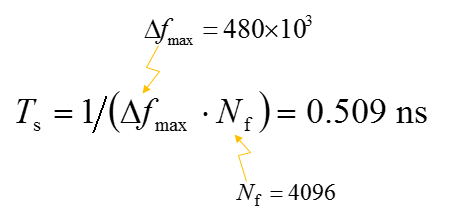As described above, in 5G/NR multiple numerologies(waveform configuration like subframe spacing) are supported and the radio frame structure gets a little bit different depending on the type of the numerology. However, regardless of numerology the length of one radio frame and the length of one subfame is same.  The length of a Radio Frame is always 10 ms and the length of a subframe is always 1 ms.

Then what should get different to accomondate the physical property of the different numerology ? The anwer is to put different number of slots within one subfame. There is another varying parameter with numerology. It is the number of symbols within a slot. However, the number of symbols within a slot does not change with the numberology, it only changes with slot configuration type. For slot configuration 0, the number of symbols for a slot is always 14 and for slot configuration 1, the number of symbols for a slot is always 7.

Now let's look into the details of radio frame structure for each numerology and slot configuration.

< Slot Configuration = 0, Numerology = 0 >

In this configuration, a subframe has only one slot in it, it means a radio frame contains 10 slots in it. The number of OFDM symbols within a slot is 14.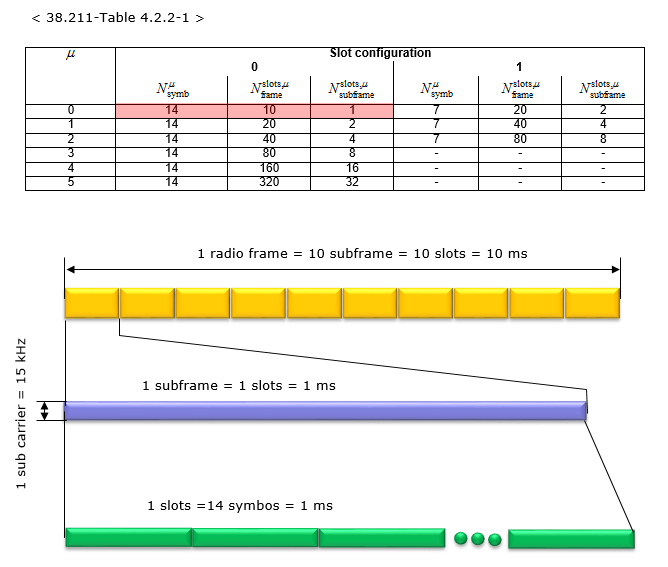< Slot Configuration = 0, Numerology = 1 >

In this configuration, a subframe has 2 slots in it, it means a radio frame contains 20 slots in it. The number of OFDM symbols within a slot is 14.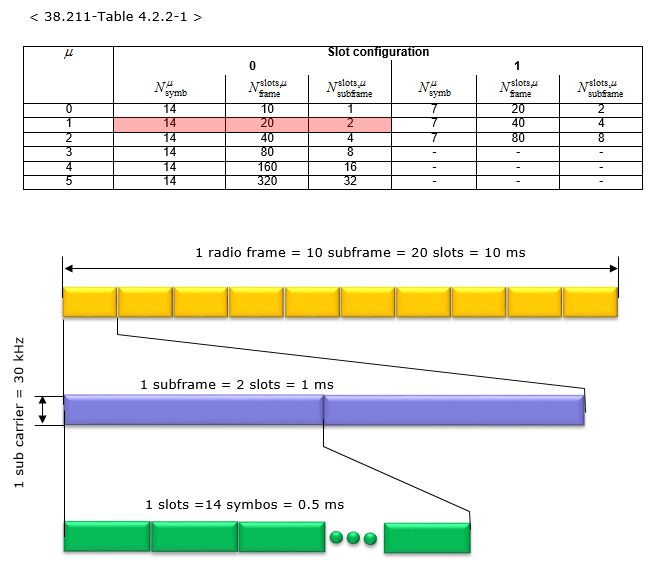< Slot Configuration = 0, Numerology = 2 >

In this configuration, a subframe has 4 slots in it, it means a radio frame contains 40 slots in it. The number of OFDM symbols within a slot is 14.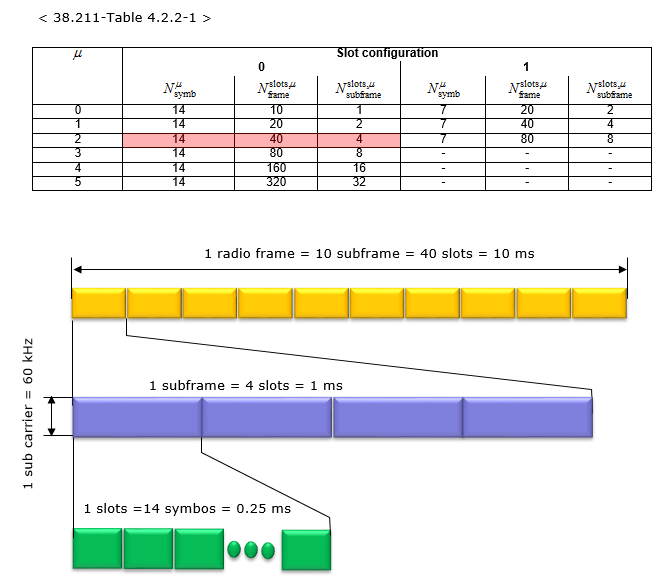< Slot Configuration = 0, Numerology = 3 >

In this configuration, a subframe has 8 slots in it, it means a radio frame contains 80 slots in it. The number of OFDM symbols within a slot is 14.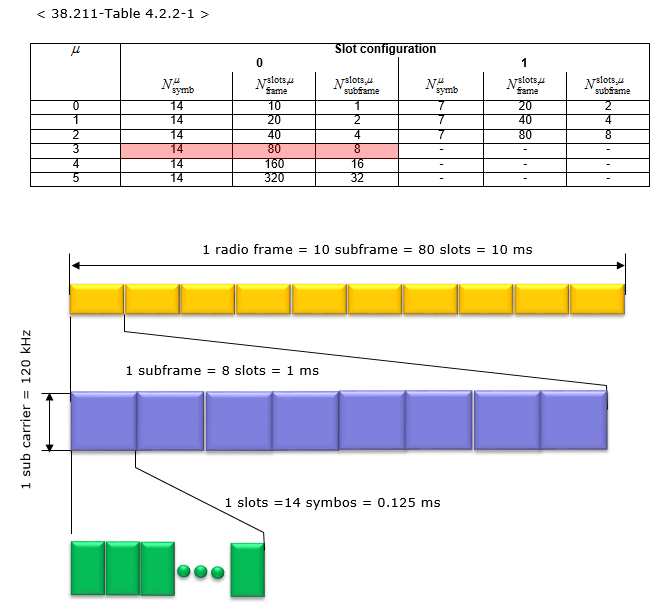< Slot Configuration = 0, Numerology = 4 >

In this configuration, a subframe has 16 slots in it, it means a radio frame contains 160 slots in it. The number of OFDM symbols within a slot is 14.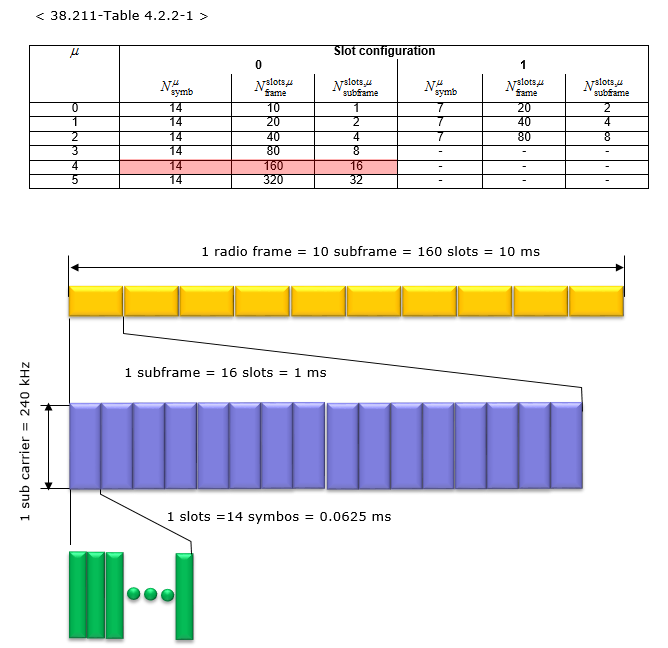< Slot Configuration = 0, Numerology = 5 >

In this configuration, a subframe has 32 slots in it, it means a radio frame contains 320 slots in it. The number of OFDM symbols within a slot is 14.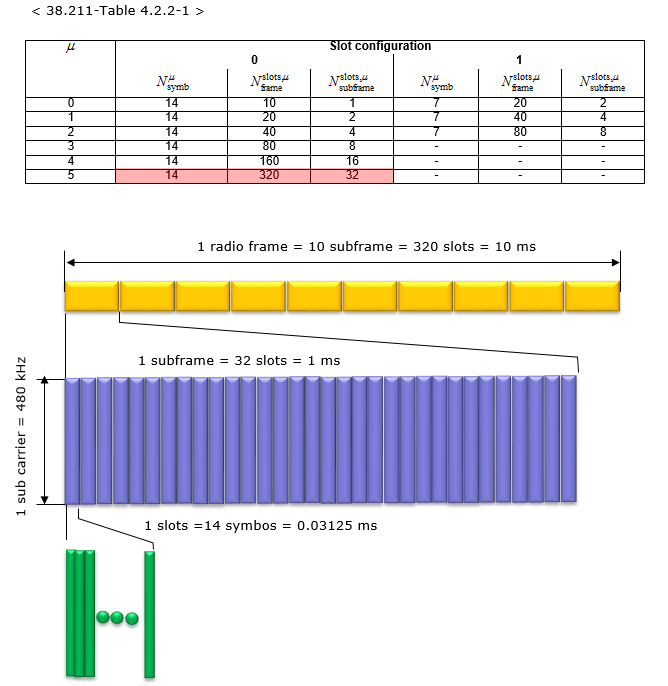< Slot Configuration = 1, Numerology = 0 >

In this configuration, a subframe has 2 slots in it, it means a radio frame contains 20 slots in it. The number of OFDM symbols within a slot is 7.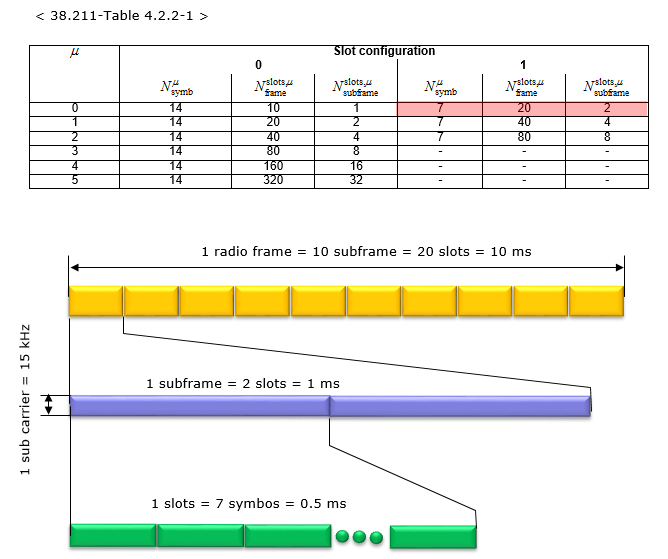< Slot Configuration = 1, Numerology = 1 >

In this configuration, a subframe has 4 slots in it, it means a radio frame contains 40 slots in it. The number of OFDM symbols within a slot is 7.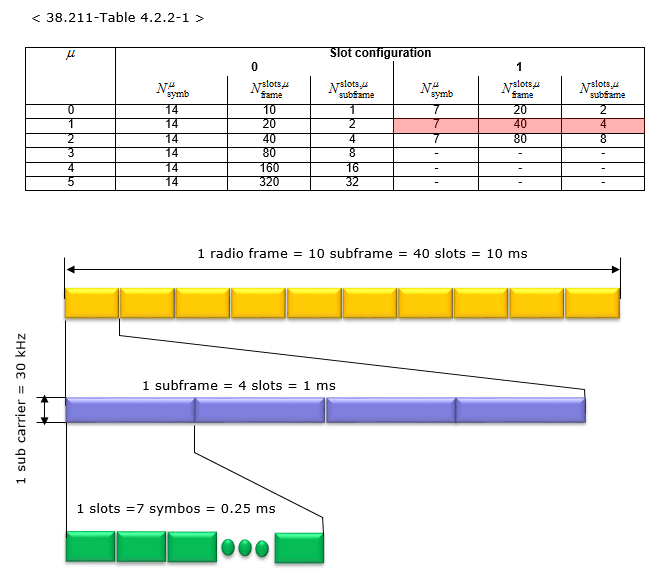< Slot Configuration = 1, Numerology = 2 >

In this configuration, a subframe has 8 slots in it, it means a radio frame contains 80 slots in it. The number of OFDM symbols within a slot is 7.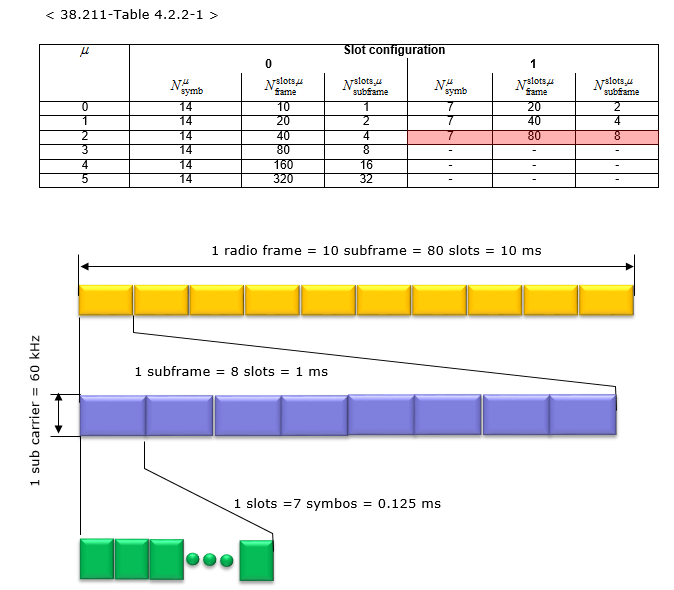Slot Format

There are a lot of different slot format defined in 38.211 (since v 2.0.0).  The concept would be similar to legacy LTE TDD Subframe configuration, but main differences from LTE TDD subframe configuration are

• in NR slot format, DL and UL assignment changes at a symbol level (in LTE TDD the UL/DL assignment is done in a subframe level)
• in NR slot format, there are much diverse patterns comparing to LTE TDD subframe configuration (Not a good news for FPGA or DSP engineers :)

<38.211 - Table 4.3.2-3: Slot formats>

 Symbol Number in a slot Format 0 1 2 3 4 5 6 7 8 9 10 11 12 13 0 D D D D D D D D D D D D D D 1 U U U U U U U U U U U U U U 2 X X X X X X X X X X X X X X 3 D D D D D D D D D D D D D X 4 D D D D D D D D D D D D X X 5 D D D D D D D D D D D X X X 6 D D D D D D D D D D X X X X 7 D D D D D D D D D X X X X X 8 X X X X X X X X X X X X X U 9 X X X X X X X X X X X X U U 10 X U U U U U U U U U U U U U 11 X X U U U U U U U U U U U U 12 X X X U U U U U U U U U U U 13 X X X X U U U U U U U U U U 14 X X X X X U U U U U U U U U 15 X X X X X X U U U U U U U U 16 D X X X X X X X X X X X X X 17 D D X X X X X X X X X X X X 18 D D D X X X X X X X X X X X 19 D X X X X X X X X X X X X U 20 D D X X X X X X X X X X X U 21 D D D X X X X X X X X X X U 22 D X X X X X X X X X X X U U 23 D D X X X X X X X X X X U U 24 D D D X X X X X X X X X U U 25 D X X X X X X X X X X U U U 26 D D X X X X X X X X X U U U 27 D D D X X X X X X X X U U U 28 D D D D D D D D D D D D X U 29 D D D D D D D D D D D X X U 30 D D D D D D D D D D X X X U 31 D D D D D D D D D D D X U U 32 D D D D D D D D D D X X U U 33 D D D D D D D D D X X X U U 34 D X U U U U U U U U U U U U 35 D D X U U U U U U U U U U U 36 D D D X U U U U U U U U U U 37 D X X U U U U U U U U U U U 38 D D X X U U U U U U U U U U 39 D D D X X U U U U U U U U U 40 D X X X U U U U U U U U U U 41 D D X X X U U U U U U U U U 42 D D D X X X U U U U U U U U 43 D D D D D D D D D X X X X U 44 D D D D D D X X X X X X U U 45 D D D D D D X X U U U U U U 46 D D D D D D X D D D D D D X 47 D D D D D X X D D D D D X X 48 D D X X X X X D D X X X X X 49 D X X X X X X D X X X X X X 50 X U U U U U U U U U U U U 51 X X U U U U U X X U U U U U 52 X X X U U U U X X X U U U U 53 X X X X U U U X X X X U U U 54 D D D D D X U D D D D D X U 55 D D X U U U U U U XU U U U U 56 D X U U U U U D X U U U U U 57 D D D D X X U D D D D X X U 58 D D X X U U U D D X X U U U 59 D X U U U U U D X U U U U U 60 D X X X X X U D X X X X X U 61 D D X X X X U D D X X X X U 62-255

Resource Grid

The resource grid for NR is defined as follows. If you just take a look at the picture, you would think it is almost identical to LTE resource grid. But the physical dimmension (i.e, subcarrier spacing, number of OFDM symbols within a radio frame) varies in NR depending on numerology.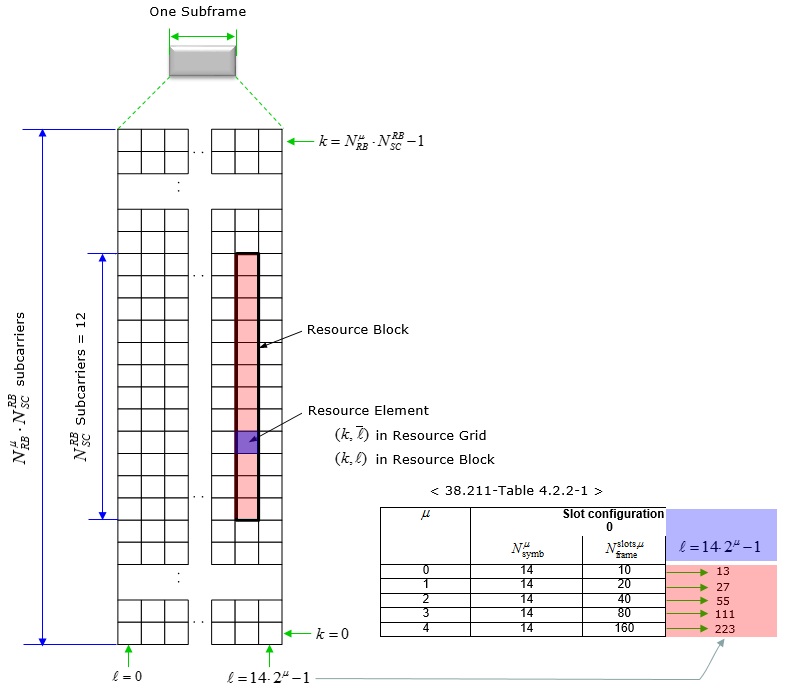The maximum and minimum number of Resource blocks for downlink and uplink is defined as below (this is different from LTE)

< 38.211-Table 4.4.2-1: Minimum and maximum number of resource blocks.>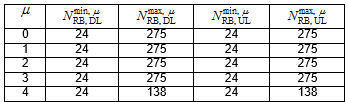Following is the table that I converted the downlink portions of Table 4.4.2-1 into frequency Bandwidth just to give you the idea on what is the maximum RF bandwidth that a UE / gNB need to support for single carrier.

 u min RB Max RB sub carrier spacing (kHz) Freq BW min (MHz) Freq BW max (MHz) 0 24 275 15 4.32 49.5 1 24 275 30 8.64 99 2 24 275 60 17.28 198 3 24 275 120 34.56 396 4 24 138 240 69.12 397.44 5 24 69 480 138.24 397.44

SS/PBCH

SS(PSS and SSS) and PBCH in NR is transmitted in the same 4 symbol block as specified in the following table.

< Frequency Domain Resource Allocation >

< 38.211- Table 7.4.3.1-1: Resources within an SS/PBCH block for PSS, SSS, PBCH, and DM-RS for PBCH >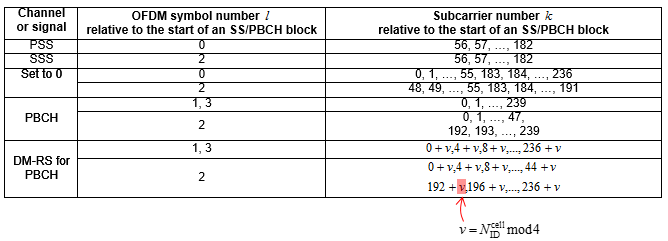This table can be represented in Resource Grid as shown below. Note that the position of PBCH DM-RS varies with v and the value v changes depending on Physical Cell ID.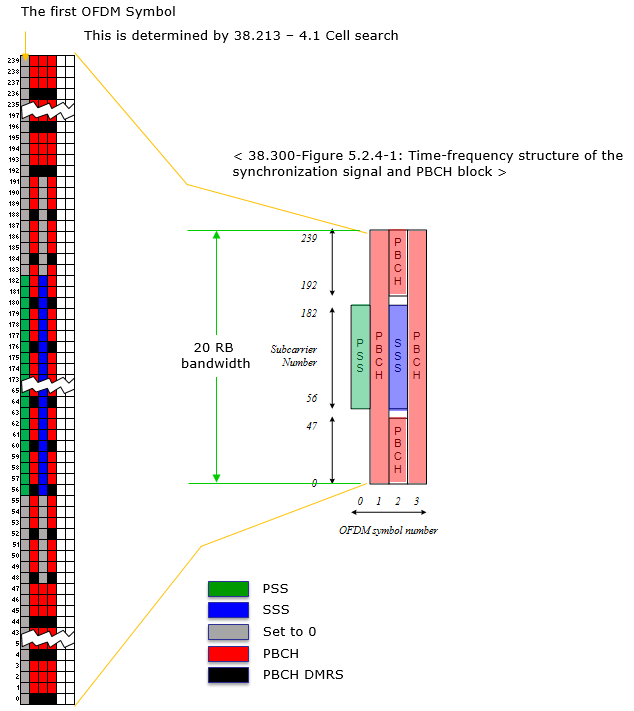< Time Domain Resource Allocation >

Following table indicates the first OFDM symbol number (s) where SS/PBCH is transmitted. This is based on 38.213 - 4.1 Cell Search

 Subcarrier Spacing OFDM Symbol (s) f <= 3 Ghz 3 Ghz < f <= 6 Ghz 6 Ghz < f Case A : 15 KHz {2,8} + 14 n n = 0,1 n = 0,1,2,3 s = 2,8,16,22 s = 2,8,16,22,30,36 Case B : 30 Khz {4,8,16,20}+28n n = 0 n = 0,1 s = 4,8,16,20 s = 4,8,16,20,32,36,      44,48 Case C : 60 Khz {2,8} + 14 n n = 0,1 n = 0,1,2,3 s = 2,8,16,22 s = 2,8,16,22,30,36 Case D : 120 Khz {4,8,16,20} + 28n n=0, 1, 2, 3, 5, 6, 7, 8, 10, 11, 12, 13, 15, 16, 17, 18 s = 4,8,16,20,      32,36,44,48,      60,64,72,76,      88,92,100,104,      144,148,156,160,      172,176,184,188,      200,204,212,216,      228,232,240,244,      284,288,296,300,      312,316,324,328,      340,344,352,356,      368,372,380,384,      424,428,436,440,      452,456,464,468,      480,484,492,496,      508,512,520,524 Case E : 240 Khz {8, 12, 16, 20, 32, 36, 40, 44} + 56n n=0, 1, 2, 3, 5, 6, 7, 8 s = 8,12,16,20,      32,36,40,44,      64,68,72,76,      88,92,96,100,      120,124,128,132,      144,148,152,156,      176,180,184,188,      200,204,208,212,      288,292,296,300,      312,316,320,324,      344,348,352,356,      368,372,376,380,      400,404,408,412,      424,428,432,436,      456,460,464,468,      480,484,488,492

Reference

 3GPP TSG RAN WG1 Meeting NR#3 : R1- 1716650 Comparison of PBCH DMRS mapping schemes

 3GPP TSG RAN WG1 Meeting NR#3 : R1-1715841 Remaining Details on PBCH design and contents

 3GPP TSG RAN WG1 Meeting AH_NR#3 : R1-1716609 - On remaining details of NR DL DMRS

 3GPP TSG RAN WG1 NR Ad-Hoc#3 : R1-1716574 - Discussion on time domain resource allocation